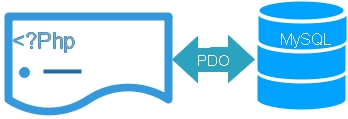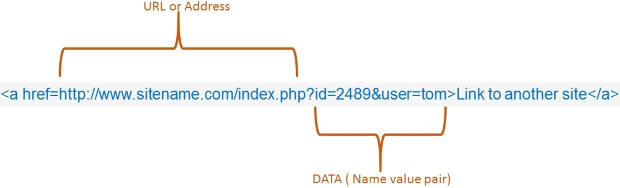# Example of SQL injection attackDisplaying records

Let us understand how code can be injected into SQL code as data through variable.
We are reading id value from string and getting the record details from the table. Our student table has one unique id for each student record. Let us first understand how data from query string is used to develop SQL and applied against any database table.This is an URL, we can pass the value of id as part of the query string ( URL ).
On the targeted page the id value will be available like this.
``\$id=\$_GET['id']// reading from URL the id value i.e 2489``
We can build a query using this variable like this.
``\$sql="SELECT * FROM student where id=\$id";``
However the URL can easily be changed by adding this data in place of the id value.
``\$id='10; DROP TABLE student--';``
The second part of the variable data saying `DROP TABLE student--` will be executed as one more query and delete the student table.
The following code will delete the student table.
``````require "config.php"; // Database Connection
\$id=\$_GET['id'];
\$id='10; DROP TABLE student--';// Injected data for id
\$sql="SELECT * FROM student where id=\$id";

\$step=\$dbo->query(\$sql);
\$row = \$step->fetch(PDO::FETCH_OBJ);
echo "<br> name =\$row->name<br>";
echo "<hr>";``````
However by using Parameterized query we can prevent the SQL injection attack. This query won’t able to delete the student table.
``````\$sql="SELECT * FROM student WHERE id=:id";
\$count=\$dbo->prepare(\$sql);
\$count->bindParam(":id",\$id,PDO::PARAM_INT,3);

if(\$count->execute()){
echo " Success <br>";
\$row = \$count->fetch(PDO::FETCH_OBJ);
print_r(\$row);
``Download Zip file to test your PHP PDO script``Subscribe to our YouTube Channel here

## Subscribe

* indicates required
Subscribe to plus2netplus2net.com